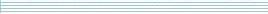| home      | people     | research     | publications    | seminars    | events     | contactCommunication Networks   | Systems Biology   | Hybrid Systems    | Machine Learning   | Dynamics & Interaction### Schools Mathematics Grand Challenge

Week four's Puzzles

Problem 7:

In this problem,the notation x^y means the number x raised to the power of y. For example,

2^3 is 2 raised to the power of 3 = 2x2x2 = 8 3^2 is 3 raised to the power of 2 = 3x3 = 9 2^5 is 2 raised to the power of 5 = 2x2x2x2x2 = 32

Also, the last digit of a positive whole number is the digit in the "ones" position, farthest to the right. For example, the last digit of

156753

is 3.

What is the last digit of

(2004)^1,000,000 + (2005)^1,000,000?

You need to give the last digit of the number you will get if you raise 2004 to the power of 1,000,000 (one million) and add it to 2005 raised to the power of 1,000,000?

You should not need to work out the actual number!!

Problem 8:

Julie has a large collection of one cent coins (and a lot of spare time!!) and wants to divide the coins into piles, each of which contains the same number of coins. First of all, she tries to put ten coins into each pile but when she comes to the last pile there are only nine coins left (so that the last pile is one cent short). She tries again, this time putting nine coins into each pile. This time, she finds that there are eight coins left over in the last pile. So she starts again, putting eight coins in each pile. Once again the same problem occurs and there are only seven coins left for the last pile. She continues in the same way trying to organise the coins into piles of 7, 6, 5, 4, 3 and 2 coins but on each occasion finds that the last pile is exactly one coin short. So she can only evenly divide the coins into piles of one coin each!

If Julie has less than 3000 coins, how many 1 cent coins does she actually have?